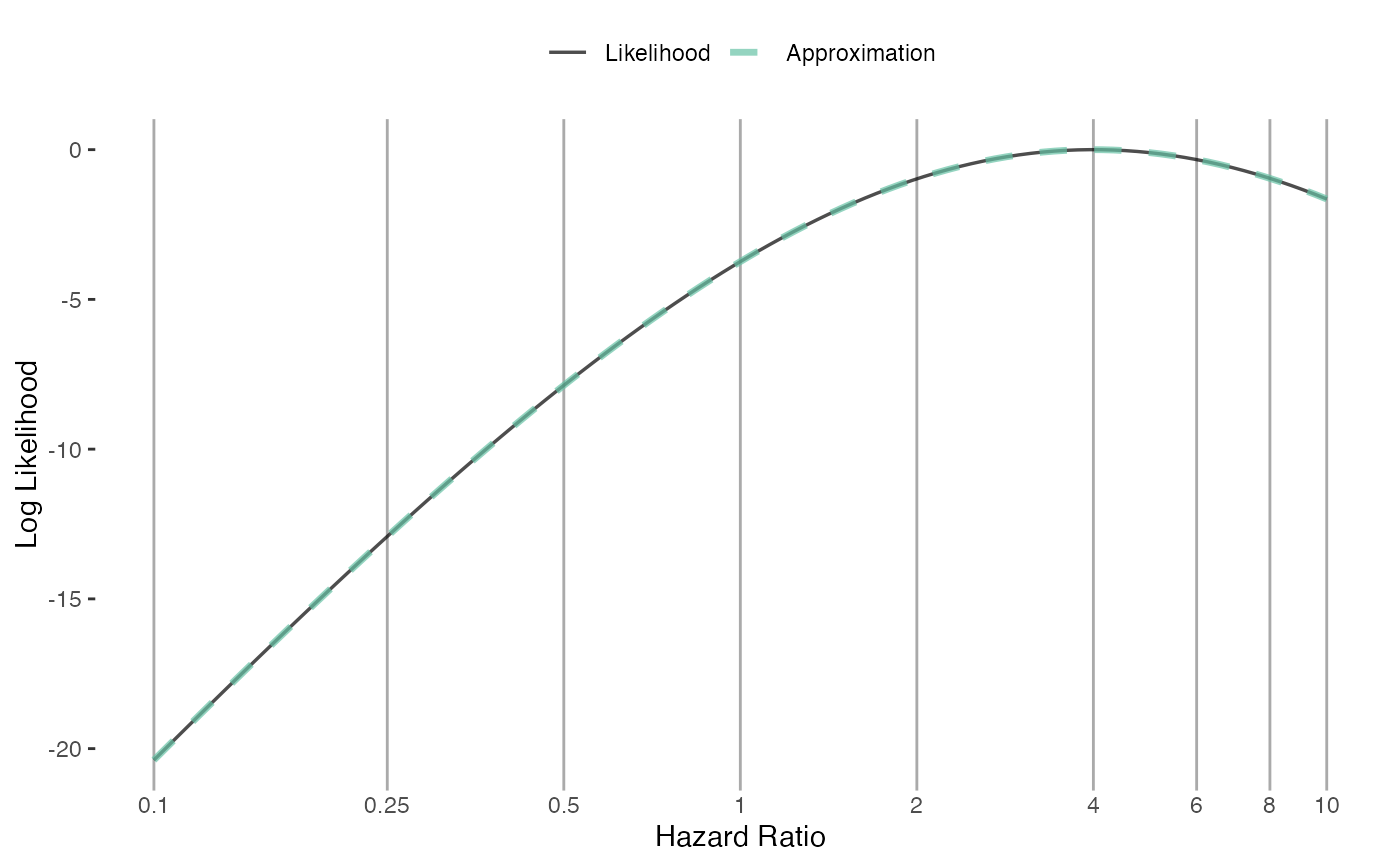Plot the likelihood approximation

plotLikelihoodFit(
approximation,
cyclopsFit,
parameter = "x",
logScale = TRUE,
xLabel = "Hazard Ratio",
limits = c(0.1, 10),
fileName = NULL
)

## Arguments

approximation

An approximation of the likelihood function as fitted using the approximateLikelihood() function.

cyclopsFit

A model fitted using the Cyclops::fitCyclopsModel() function.

parameter

The parameter in the cyclopsFit object to profile.

logScale

Show the y-axis on the log scale?

xLabel

The title of the x-axis.

limits

The limits on the x-axis.

fileName

Name of the file where the plot should be saved, for example 'plot.png'. See the function ggplot2::ggsave in the ggplot2 package for supported file formats.

## Value

A Ggplot object. Use the ggplot2::ggsave function to save to file.

## Details

Plots the (log) likelihood and the approximation of the likelihood. Allows for reviewing the approximation.

## Examples

# Simulate a single database population:
population <- simulatePopulations(createSimulationSettings(nSites = 1))[]

# Approximate the likelihood:
cyclopsData <- Cyclops::createCyclopsData(Surv(time, y) ~ x + strata(stratumId),
data = population,
modelType = "cox"
)
cyclopsFit <- Cyclops::fitCyclopsModel(cyclopsData)
approximation <- approximateLikelihood(cyclopsFit, parameter = "x", approximation = "custom")

plotLikelihoodFit(approximation, cyclopsFit, parameter = "x")
#> Detected data following custom parameric distribution